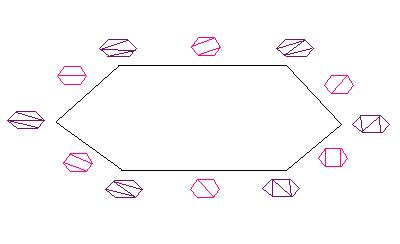Another interesting sequence of polytopes is the cyclohedra, but these are thought of as a module for an operad. The $n$th cyclohedron is a $K( \pi , 1)$ space, which means that there exists a group $\pi$ such that the first homotopy group of the space is $\pi$ and all other homotopy groups are trivial. This is mentioned in one of Devadoss's classic papers, which explains the labelling on the 2-cyclohedron, namely the hexagon.Note that hexagons with chords are also used to label the three dimensional Stasheff associahedron, but here only centrally symmetric chorded polygons appear. An appendix in a paper by Markl explains how this hexagon is turned into a triangle by shrinking down three of the sides. In general, there is a process for turning cyclohedra into regular simplices. Note that the cyclohedra are sometimes named after their inventors, Bott and Taubes.